RSWebinar: Designing Cost-Effective Ground Improvement Solutions with Settle3 | June 7th, 2023 Register Now

# Cohesionless

The following properties are required or available for all cohesionless soil layers:

• Reduction Factors (%) – percent decrease in computed unit resistance for layer
• Skin Resistance Loss
• End Bearing Loss
• Unit Resistance Limits – maximum value of unit resistance
• Skin Friction Limit
• End Bearing Limit
The skin friction resistance is capped by 5% of the entered Concrete Cylinder Strength in the pile properties.

The following parameters are required for each method as described below. For more information see Bored Capacity Theory Manual.

## Ks-Delta/NQ Method

Skin friction parameters:

• Skin Friction Angle –specify or compute from SPT Table as described in Interpretation of SPT N Values from Table section
• OCR - over consolidation ratio
• Ks/Ko - ratio between soil-pile and at-rest coefficient of lateral earth pressure
• Delta/Phi (δ/ϕ') - ratio between soil-pile interface and soil friction angle

The ultimate unit skin (frictional) resistance (fsult) is computed as follows:Where:End bearing parameters:

• End Bearing Angle - specify or compute from SPT Table as described in Interpretation of SPT N Values from Table section
• Nq - bearing capacity factor inputted or computed automatically based on end bearing friction angle

Nq may be found from various literature where one of most common is from Berezantzef presented by Polous and Davis (1980) as shown below. This graph is used for the Auto Nq option. It is advised to decrease the entered angle of internal friction by 2 to 3 degrees before using the graph. See the theory manual for more details about N q computation.The bearing capacity factor, Nq from Berezantzef.

The ultimate unit end (base) resistance (qb,ult) is computed as follows: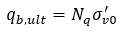Where:## SPT-AASHTO Method

Skin friction and end bearing parameters:

• SPT Table - specify SPT N values with depth

The ultimate unit skin (frictional) resistance (fs,ult) in kPa units is computed as follows: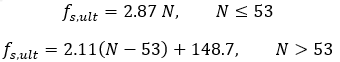The ultimate unit end (base) resistance (qb,ult) in kPa units is computed as follows:See Interpretation of SPT N Values from Table section for more details.

## SPT-User Factor Method

Skin friction and end bearing parameters:

• SPT Table - specify SPT N values with depth

Skin friction parameters:

• a and b parameters

The ultimate unit skin (frictional) resistance (fs,ult) is computed as follows:

fs,ult = a + b N

End bearing parameters:

• c and d parameters

The ultimate unit end (base) resistance (qb,ult) is computed as follows:

qb,ult = c + d N

See Interpretation of SPT N Values from Table section for more details.

## Beta/NQ Method

Skin Friction Parameters:

• Beta ( β )

The ultimate unit skin (frictional) resistance (fs,ult) is computed as follows: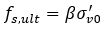Where: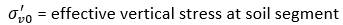End bearing parameters:

• End Bearing Angle - specify or compute from SPT Table as described in Interpretation of SPT N Values from Table section
• Nq - bearing capacity factor inputted or computed automatically based on end bearing friction angle

Nq may be found from various literature where one of most common is from Berezantzef presented by Polous and Davis (1980) as shown below. This graph is used for the Auto Nq option. It is advised to decrease the angle of internal friction by 2 to 3 degrees before using the graph. See the theory manual for more details about N q computation.The bearing capacity factor, Nq from Berezantzef.

The ultimate unit end (base) resistance (qb,ult) is computed as follows:Where: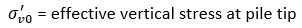## Interpretation of SPT N Values from Table

### AVERAGING SPT N VALUES

For skin segments, the SPT N value is taken at the depth of the segment. Therefore, each skin segment has an assigned SPT N value. In the case of Ks-Delta method, each skin segment will then have its own computed friction angle. For the end bearing segment, such as in the Nq method, the N value is the average from one diameter above the tip depth to two diameters below the tip depth. For non-circular piles, the shortest dimension of the pile at the tip depth is used instead of the diameter for determining the range of values in the average. A weighted average is used based on the N value and its depth.

### FRICTION ANGLE FROM SPT VALUES

There is an option to specify SPT N values with depth in order to calculate the friction angle for some methods mentioned above. RSPile uses the relationship between standard penetration test values and angle of internal friction (ϕ' ) as presented by Peck, Hanson, and Thornburn (1974).Note that the entered N values are used without correction to obtain N60.